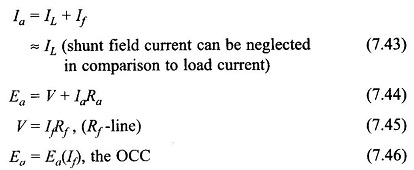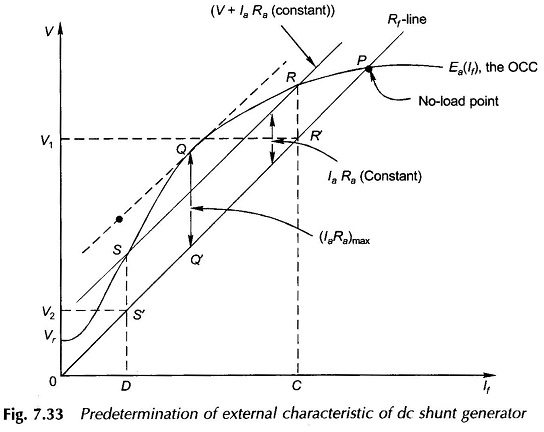## External Characteristics of DC Shunt Generator:

The External Characteristics of DC Shunt Generator can be predetermined from a knowledge of the OCC and armature and field resistances (which can be determined by simple dc test), without actually performing the load test which in fact is not feasible for a large generator. Assume for the time being that the armature reaction voltage drop is negligible.Because of the nonlinearity (saturation in the OCC), the solution of Eqs (7.44)-(7.46) to determine the terminal voltage for given Rf and laRa can be carried out graphically as shown in Fig. 7.33. For given laRa, Eq. (7.44) is represented by a straight line parallel to the Rf line and above it by vertical intercept of IaRa. The intersection of this line with the OCC gives two solution points R and S for the induced emf.

For these solution points the two terminal voltage values V1 and V2 can be read from the Rline corresponding to points R’ and S’. Maximum possible armature current corresponds to point Q at which a line parallel to the Rf line is tangent to the OCC. Corresponding to each solution point, values of V and If can be read and IL = Ia + If,can be calculated and subsequently the External Characteristics of DC Shunt Generator plotted as shown in Fig. 7.33. The short-circuit point on the external characteristic corresponds to IaRa = Vr, the residual voltage.

The voltage drop of armature reaction origin can be accounted for by modifying the OCC empirically.

Scroll to Top